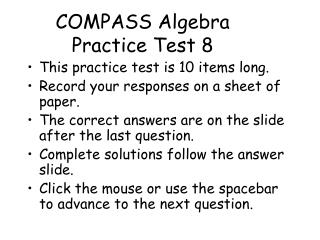DownloadDownload PresentationCOMPASS Algebra Practice Test 8

COMPASS Algebra Practice Test 8

Télécharger la présentationCOMPASS Algebra Practice Test 8

- - - - - - - - - - - - - - - - - - - - - - - - - - - E N D - - - - - - - - - - - - - - - - - - - - - - - - - - -
Presentation Transcript

1. COMPASS AlgebraPractice Test 8 • This practice test is 10 items long. • Record your responses on a sheet of paper. • The correct answers are on the slide after the last question. • Complete solutions follow the answer slide. • Click the mouse or use the spacebar to advance to the next question.

2. 1. What are the solutions to the quadratic equation

3. 2. If for all x and y, , then

4. 3. For all

5. 5. If then

6. 6. For all

7. 7. Simplify

8. 8. Simplify

9. 9. If the ratio of 4 to b is 5 to 7 then b = ?

10. 10. Which equation best describes this graph?

11. C A B A B D B B B C Answers Algebra Practice Test 8

12. 1. What are the solutions to the quadratic equation Factor. You need two numbers that will multiply to 36 and add to -15. + 37 20 15 13 12 Now use the zero factor property. Answer C

13. 2. If for all x and y, , then Solve for b. Add b to both sides. Answer A

14. 3. For all Factor. Cancel. Answer B

15. 4. What is the value of the expression when Substitute and simplify. Answer A

16. 5. If then Radical Equation First isolate the radical. Subtract 4 from both sides. Square both sides to get rid of the radical. Subtract 5 from both sides. Divide by 5. Answer B

17. 6. For all Find the square root of x9. Each pair of (xx) inside represents one x outside. Choice D is the only answer that matches this result.

18. When raising a power to a power multiply the exponents. 7. Simplify Answer B

19. Simplify the radicals. 8. Simplify The common denominator is 3 Answer B

20. 9. If the ratio of 4 to b is 5 to 7 then b = ? Write the proportion as two equal fractions. Cross multiply. Divide by 5. Answer B

21. 10. Which equation best describes this graph? The y-intercept of the graph is (0, 3). This eliminates choices A and E. The point (0, 3) works in B, C, and D.

22. 10. Which equation best describes this graph? Try the point (-1, 4) in B. This eliminates choice B. Try the point (-1, 4) in D. This eliminates choice D.

23. 10. Which equation best describes this graph? Try the point (-1, 4) in C. Answer C